Advanced Database Management System - Tutorials and Notes: Probabilistic Context Free Grammar PCFG - How to derive probabilities for production rules from treebank

## Search Engine

Please visit, subscribe and share 10 Minutes Lectures in Computer Science

# Probabilistic Context Free Grammar (PCFG) - How to calculate probabilities for production rules from Treebank?

## PCFG Solved Exercise

Question:
Consider the following 3 trees as a Treebank and derive a Probabilistic Context Free Grammar(PCFG) from this Treebank. [Derive the probabilities of production rules]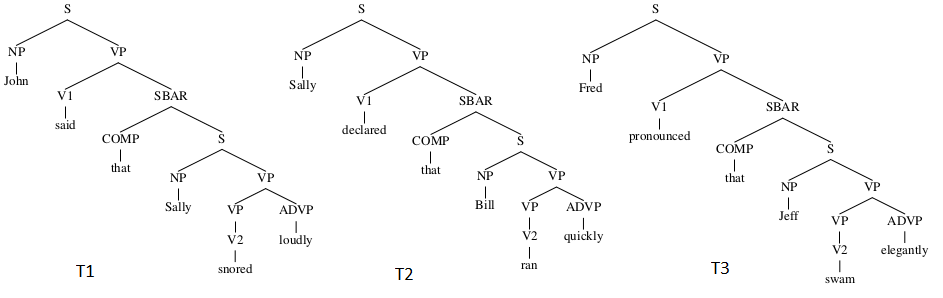Solution:
Let us assume that the given Treebank is the training corpus. It consists of common start symbol S, set of non-terminals, set of terminal symbols and production rules. Each rule derives a non-terminal to another set of non-terminals or combination of terminals and non-terminals. PCFG will consist of these rules with the probability for each rule.
The probability estimate for a rule A → s can be calculated using Maximum Likelihood estimate. Here, s is a sequence of terminals and non-terminals. It is from (T U V)*, infinite set of strings. [Refer here for formal definition of CFG]
The probability estimate of a production rule can be written as,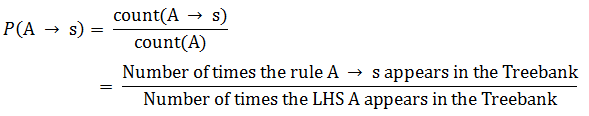Let us use this equation to derive the probabilities;
1. If you look at the Treebank, the common start symbol S is derived to NP and VP. This is the rule S → NP VP.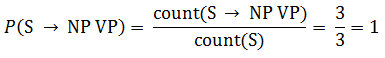In the given Treebank, the rule S → NP VP appears 3 times and S on the Left Hand Side (LHS) appears 3 times.

2. V1 and SBAR derived from VP. Hence, VP → V1 SBAR.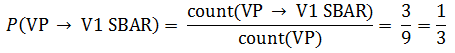3. Next rule, SBAR → COMP S.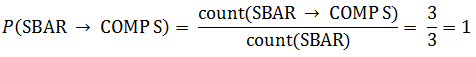4. Let us choose one rule that has only the terminal symbol on its RHS. For example, the rule, NP → John is one such rule (We refer it as lexicon).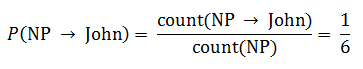The other probabilities can be calculated as shown above. As a result, we get the grammar and the corresponding probabilities for the PCFG as follows;

 Rule Probability S → NP VP SBAR → COMP S COMP → that VP → V1 SBAR | VP ADVP | V2 NP → Sally NP → John|Bill|Fred|Jeff V1 → said|declared|pronounced V2 → snored|ran|swam ADVP → loudly | quickly |elegantly 1 1 1 1/3 1/3 1/6 1/3 1/3 1/3

**********

• Go to NLP Solved Exercise page